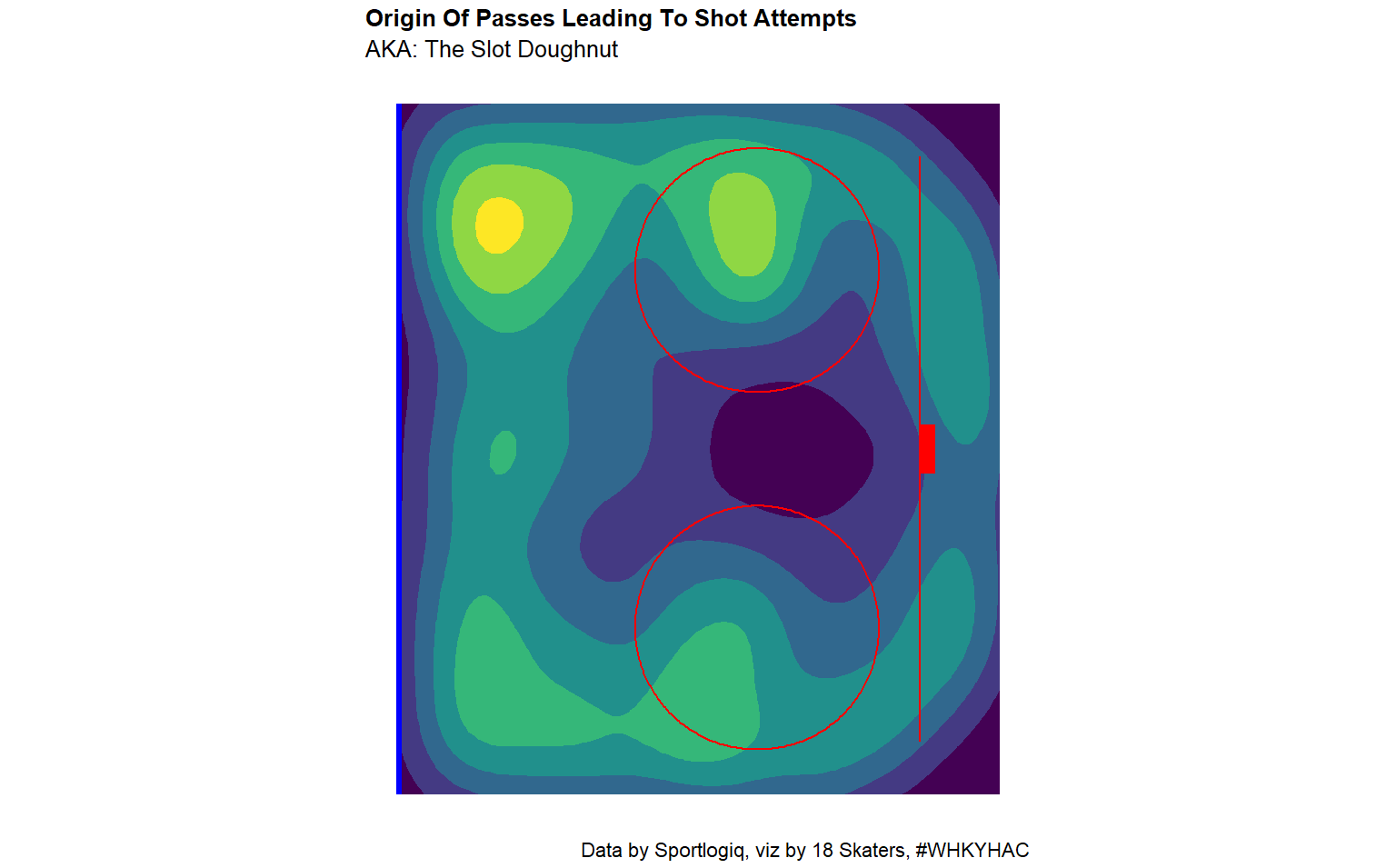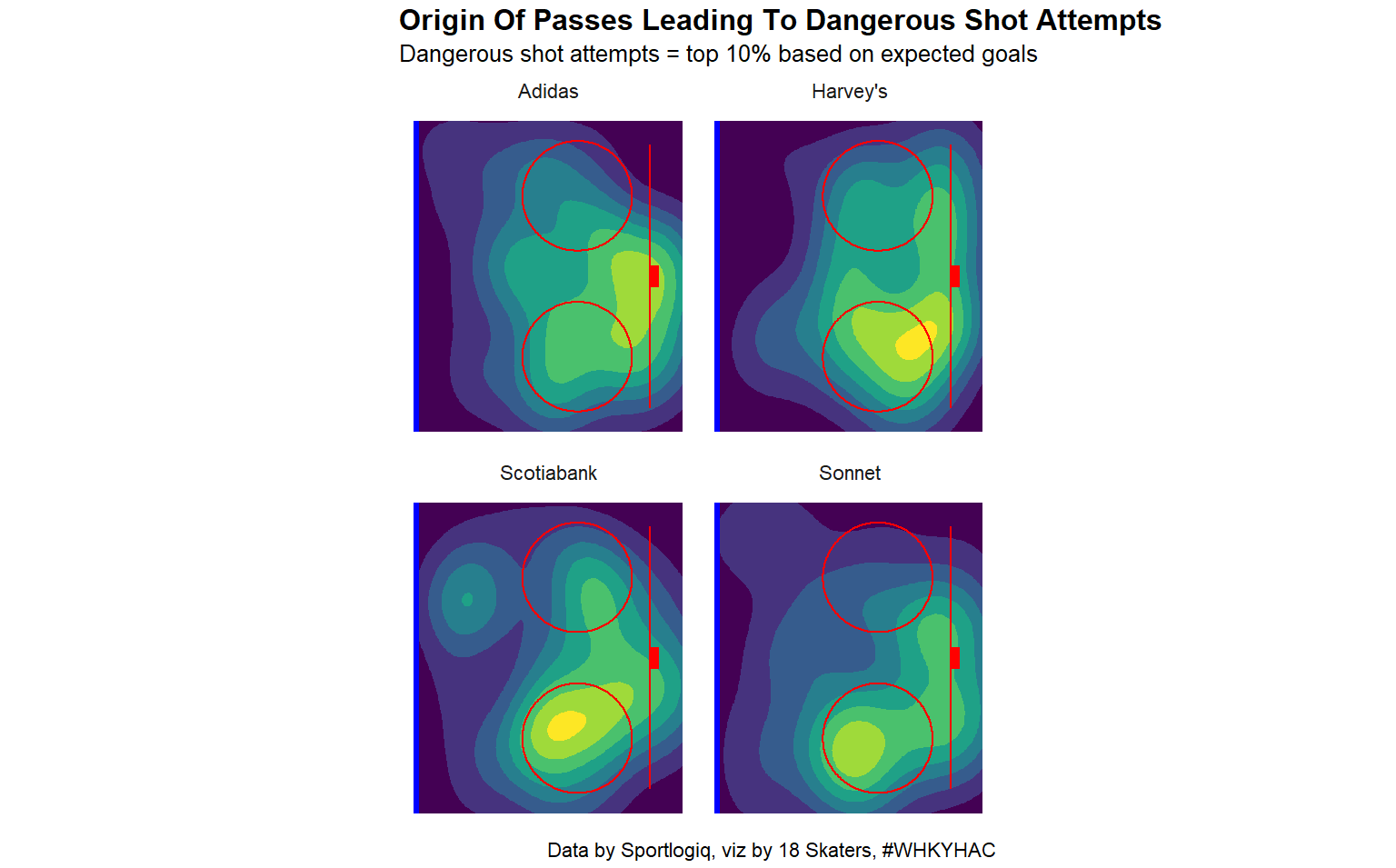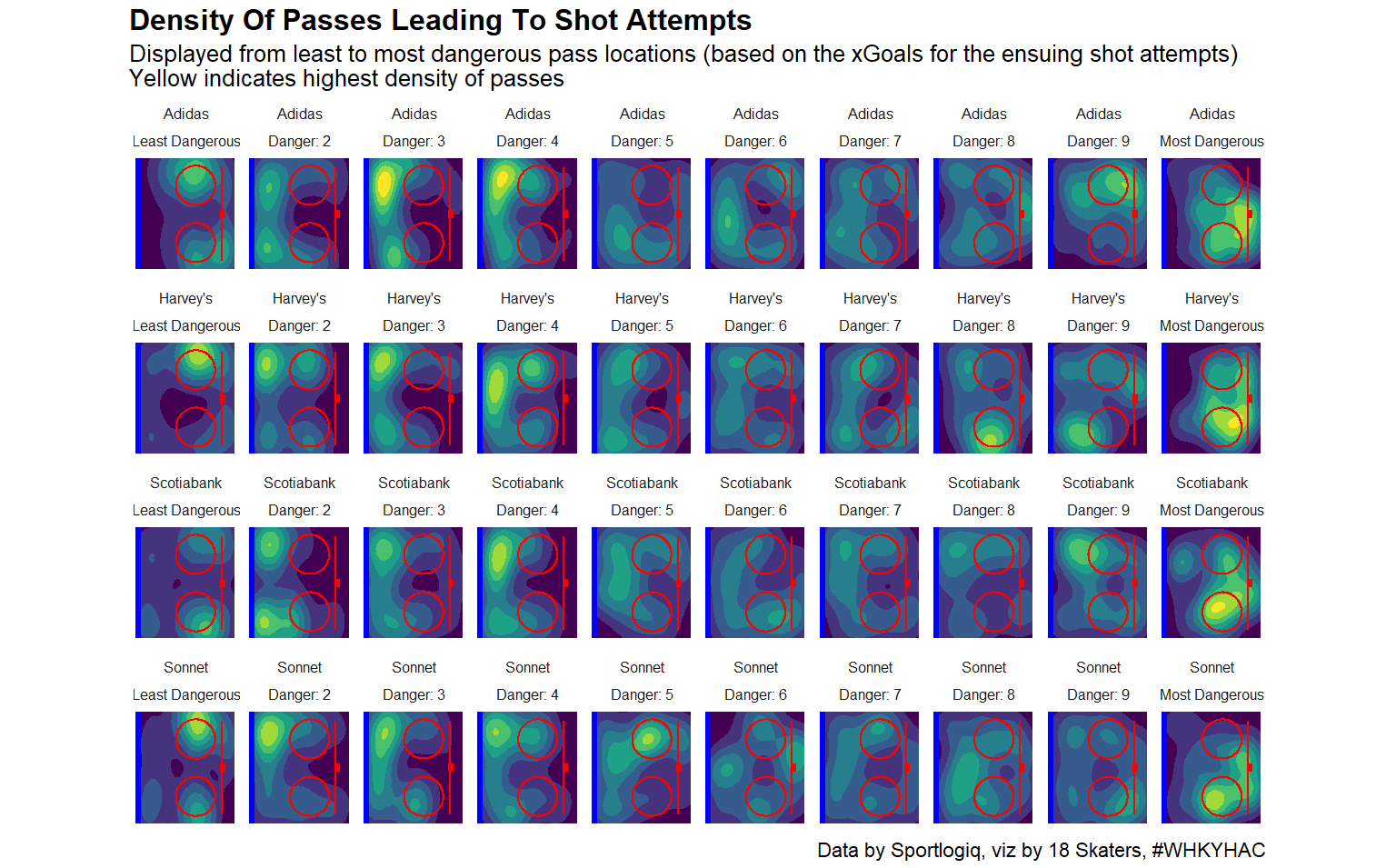### WHKYHAC: O-Zone Pass Origins Viz

WHKYHAC and Sportlogiq announced a visualization competition using data from the 2023 Season of the Professional Women’s Hockey Players Association. The entry deadline for the competition is July 2, 2023. Prior to that deadline I’ll post some “trial” data visualizations here.

## Today’s Data Viz

This post is about O-Zone passing data. I’ll look at all passes that led directly to shot attempts, then I’ll look at those passes based on their danger level (on a team-by-team basis).

## Pass Locations With xGoal and Team Analysis

The first viz shows the origin of any pass that led directly to a shot attempt in the O-Zone. It’s a density plot and the yellow areas show the highest density of passes that led to shots.

Open the image in a new tab if you want to see a larger version.There weren’t many passes originating from the slot. Presumably players were shooting the puck instead of passing it from that location.

The next viz shows only passes that led to the most dangerous shot attempts. The “danger level” of a shot attempt is simply it’s expected goal value. This viz shows the 10% most dangerous passes and is split by team.

Open the image in a new tab if you want to see a larger version.There are some similarities between the teams. Most of the dangerous pass attempts came from either the “bottom” circle (attacking from the right) or from around the goal line. Team Scotiabank shows a blip from the left defense spot.

The final viz shows the danger level of each team’s passes split into deciles. In other words, the passes are split into 10% chunks based on the xGoal values of the resulting shot attempts. While the trend is not uniform across all teams, the lower danger passes migrate from the “top” of the plot and around the point before snapping to the highest danger areas on the “bottom” circle and along the goal line.

Open the image in a new tab if you want to see a larger version.## The Code

Here’s the code for this data viz.

``````# SETUP ########################################################################

setwd("~/18_skaters/r_studio/whkyhac")

library(tidyverse)
library(stringr)
library(lubridate)
library(ggforce)

locale = locale(encoding = "ISO-8859-1"))

# EXPLORE DATA #################################################################

#print(str(raw_pbp_data))

# Players

player_names <- unique(raw_pbp_data\$player)

# Events

event_names <- unique(raw_pbp_data\$eventname)

event_outcomes <- unique(raw_pbp_data\$outcome)

event_types <- unique(raw_pbp_data\$type)

# Strength states

strength_states <- unique(raw_pbp_data\$strengthstate)

# CLEAN AND MANIPULATE DATA (AREAS OF INTEREST ONLY) ###########################

clean_pbp_data <- raw_pbp_data

# Fix name for Kristin O’Neill

clean_pbp_data\$player <- str_replace_all(clean_pbp_data\$player, "\\031", "’")

clean_pbp_data\$game_id <- paste(clean_pbp_data\$game,
clean_pbp_data\$date)

clean_pbp_data\$event_id <- seq(1:length(clean_pbp_data\$seasonstage))

# Add empty_net to opposing_goalie variable

clean_pbp_data\$opposing_goalie <- ifelse(
is.na(clean_pbp_data\$opposing_goalie),
"empty_net",
clean_pbp_data\$opposing_goalie)

# Reorganize a little

clean_pbp_data <- select(clean_pbp_data, c(30:31,1:29))

# EXPLORE SHOT ASSIST DATA #####################################################

# Get shots and corresponding event ids

shot_data <- filter(clean_pbp_data,
eventname == "shot")

shot_ids <- shot_data\$event_id

# Get prior event ids

prior_event_ids <- shot_ids - 1

# Tag prior events

clean_pbp_data <- mutate(clean_pbp_data,
prior_event = ifelse(
event_id %in% prior_event_ids,
TRUE,
FALSE))

# Summarize prior events

prior_events_summary <- filter(clean_pbp_data,
prior_event == TRUE) %>%
group_by(eventname,
type,
outcome) %>%
summarise(sum = n()) %>%
arrange(desc(sum)) %>%
ungroup()

# Plot pass receptions

prior_event_reception_data <- filter(clean_pbp_data,
prior_event == TRUE,
eventname == "reception",
outcome == "successful")

plot_prior_event_reception <- ggplot(prior_event_reception_data) +
colour = type)) +
theme_minimal()

#plot_prior_event_reception

# Tag events prior to reception

reception_ids <- prior_event_reception_data\$event_id

prior_event_ids_receptions <- reception_ids - 1

clean_pbp_data <- mutate(clean_pbp_data,
prior_event_reception = ifelse(
event_id %in% prior_event_ids_receptions,
TRUE,
FALSE))

prior_events_receptions_summary <- filter(clean_pbp_data,
prior_event_reception == TRUE) %>%
group_by(eventname,
type,
outcome) %>%
summarise(sum = n()) %>%
arrange(desc(sum)) %>%
ungroup() # This is uglier than I had hoped

# Get xA1 FOR SHOT ATTEMPT ASSISTS DATA ########################################

# Target pass receptions that were immediately followed by shot attempts in the o-zone (pass > reception > shot)

shot_attempt_data <- filter(clean_pbp_data,
eventname == "shot",

shot_attempt_ids <- shot_attempt_data\$event_id

pass_reception_data <- filter(clean_pbp_data,
event_id %in% (shot_attempt_ids - 1),
eventname == "reception",
outcome == "successful",

pass_reception_ids <- pass_reception_data\$event_id

eligible_shot_attempt_ids <- pass_reception_ids + 1

# Loop through data looking for a pass one event prior to a reception

loop_list_1 <- list()

for (i in 1:length(pass_reception_ids)) {

loop_data <- clean_pbp_data %>%
filter(event_id == pass_reception_ids[i] - 1)

loop_data <- mutate(loop_data,
pass_1 = ifelse(
eventname == "pass",
TRUE,
FALSE))

loop_list_1[[i]] <- loop_data
}

pass_1_event_prior_data <- bind_rows(loop_list_1)

pass_1_event_prior_data <- select(pass_1_event_prior_data,
event_id,
pass_1)

# Loop through data looking for a pass two events prior to a reception

loop_list_2 <- list()

for (i in 1:length(pass_reception_ids)) {

loop_data <- clean_pbp_data %>%
filter(event_id == pass_reception_ids[i] - 2)

loop_data <- mutate(loop_data,
pass_2 = ifelse(
eventname == "pass",
TRUE,
FALSE))

loop_list_2[[i]] <- loop_data
}

pass_2_event_prior_data <- bind_rows(loop_list_2)

pass_2_event_prior_data <- select(pass_2_event_prior_data,
event_id,
pass_2)

# Loop through data looking for a pass three events prior to a reception

loop_list_3 <- list()

for (i in 1:length(pass_reception_ids)) {

loop_data <- clean_pbp_data %>%
filter(event_id == pass_reception_ids[i] - 3)

loop_data <- mutate(loop_data,
pass_3 = ifelse(
eventname == "pass",
TRUE,
FALSE))

loop_list_3[[i]] <- loop_data
}

pass_3_event_prior_data <- bind_rows(loop_list_3)

pass_3_event_prior_data <- select(pass_3_event_prior_data,
event_id,
pass_3)

# Eliminate earlier passes for pass_3

pass_1_ids <- filter(pass_1_event_prior_data,
pass_1 == TRUE)
pass_1_ids <- pass_1_ids\$event_id

pass_3_event_prior_data\$pass_3 <- ifelse(
pass_3_event_prior_data\$event_id %in% (pass_1_ids - 2),
FALSE,
pass_3_event_prior_data\$pass_3)

# Loop through data looking for a pass four events prior to a reception

loop_list_4 <- list()

for (i in 1:length(pass_reception_ids)) {

loop_data <- clean_pbp_data %>%
filter(event_id == pass_reception_ids[i] - 4)

loop_data <- mutate(loop_data,
pass_4 = ifelse(
eventname == "pass",
TRUE,
FALSE))

loop_list_4[[i]] <- loop_data
}

pass_4_event_prior_data <- bind_rows(loop_list_4)

pass_4_event_prior_data <- select(pass_4_event_prior_data,
event_id,
pass_4)

# Eliminate earlier passes for pass_4

pass_2_ids <- filter(pass_2_event_prior_data,
pass_2 == TRUE)
pass_2_ids <- pass_2_ids\$event_id

pass_4_event_prior_data\$pass_4 <- ifelse(
pass_4_event_prior_data\$event_id %in% (pass_1_ids - 3),
FALSE,
pass_4_event_prior_data\$pass_4)

pass_4_event_prior_data\$pass_4 <- ifelse(
pass_4_event_prior_data\$event_id %in% (pass_2_ids - 2),
FALSE,
pass_4_event_prior_data\$pass_4)

# Join the loop data to the play-by-play data

clean_pbp_data <- clean_pbp_data %>%
left_join(pass_1_event_prior_data,
by = "event_id") %>%
left_join(pass_2_event_prior_data,
by = "event_id") %>%
left_join(pass_3_event_prior_data,
by = "event_id") %>%
left_join(pass_4_event_prior_data,
by = "event_id")

# Filter for target data

target_data <- filter(clean_pbp_data,
event_id %in% eligible_shot_attempt_ids |
event_id %in% pass_reception_ids |
pass_1 == TRUE |
pass_2 == TRUE |
pass_3 == TRUE |
pass_4 == TRUE)

# Check for pass > reception > shot pattern in the target data
# This should print a repeating pattern of "1 2 3"

pattern_summary <- target_data %>%
group_by(eventname) %>%
summarise(count = n()) %>%
ungroup()

options(max.print=10000)
#match(target_data\$eventname,
#c("pass", "reception", "shot"))
options(max.print=1000)

# Fill xG data

target_data <- target_data %>%
fill(xg_all_attempts, .direction = "up")

# PLOT THE RESULTS #############################################################

# Tidy the data (selecting only O-Zone passes)

plot_data_ozone_passes <- filter(target_data,
eventname == "pass",

plot_data_ozone_passes <- select(plot_data_ozone_passes,
teamname,
eventname,
outcome,
type,
xg_all_attempts)

# According to the Data Dictionary I need to multiply yadjcoord by -1

# Density plot of all pass origins

all_pass_density_plot <- ggplot(plot_data_ozone_passes) +
geom_segment(aes(x = 89,
y = -36,
xend = 89,
yend = 36),
colour = "red",
alpha = 0.5,
linewidth = 0.8) +
geom_segment(aes(x = 25,
y = -42.5,
xend = 25,
yend = 42.5),
colour = "blue",
alpha = 0.5,
linewidth = 2) +
geom_segment(aes(x = 90,
y = 3,
xend = 90,
yend = -3),
linewidth = 4.8,
alpha = 0.5,
colour = "red") +
geom_circle(aes(x0 = 69,
y0 = 22,
r = 15),
colour = "red",
alpha = 0.5,
linewidth = 0.8) +
geom_circle(aes(x0 = 69,
y0 = -22,
r = 15),
colour = "red",
alpha = 0.5,
linewidth = 0.8) +
theme_minimal() +
theme(panel.grid = element_blank(),
axis.title = element_blank(),
axis.text = element_blank(),
plot.title.position = "plot",
plot.caption.position = "plot",
legend.position = "none",
plot.title = element_text(size = 16,
face = "bold"),
plot.subtitle = element_text(size = 16),
plot.caption = element_text(size = 14)) +
labs(title = "Origin Of Passes Leading To Shot Attempts",
subtitle = "AKA: The Slot Doughnut",
caption = "Data by Sportlogiq, viz by 18 Skaters, #WHKYHAC") +
coord_fixed()
#all_pass_density_plot

# Density plot of top 10% pass origins (based on xG) with team facet_wrap

xg_deciles <- ntile(plot_data_ozone_passes\$xg_all_attempts, 10)

plot_data_ozone_passes\$decile <- xg_deciles

plot_data_decile_shrink <- plot_data_ozone_passes %>%
filter(decile == 10)

top_10_pass_density_team_plot <- ggplot(plot_data_decile_shrink) +
geom_segment(aes(x = 89,
y = -36,
xend = 89,
yend = 36),
colour = "red",
alpha = 0.5,
linewidth = 0.8) +
geom_segment(aes(x = 25,
y = -42.5,
xend = 25,
yend = 42.5),
colour = "blue",
alpha = 0.5,
linewidth = 2) +
geom_segment(aes(x = 90,
y = 3,
xend = 90,
yend = -3),
linewidth = 3.5,
alpha = 0.5,
colour = "red") +
geom_circle(aes(x0 = 69,
y0 = 22,
r = 15),
colour = "red",
alpha = 0.5,
linewidth = 0.8) +
geom_circle(aes(x0 = 69,
y0 = -22,
r = 15),
colour = "red",
alpha = 0.5,
linewidth = 0.8) +
theme_minimal() +
theme(panel.grid = element_blank(),
axis.title = element_blank(),
axis.text = element_blank(),
plot.title.position = "plot",
plot.caption.position = "plot",
legend.position = "none",
plot.title = element_text(size = 20,
face = "bold"),
plot.subtitle = element_text(size = 16),
plot.caption = element_text(size = 14),
strip.text = element_text(size = 14)) +
labs(title = "Origin Of Passes Leading To Dangerous Shot Attempts",
subtitle = "Dangerous shot attempts = top 10% based on expected goals",
caption = "Data by Sportlogiq, viz by 18 Skaters, #WHKYHAC") +
coord_fixed() +
facet_wrap(vars(teamname))
#top_10_pass_density_team_plot

# Density plot of pass origins with team and decile facet_wrap

decile_labels <- c("Least Dangerous", "Danger: 2", "Danger: 3", "Danger: 4", "Danger: 5", "Danger: 6", "Danger: 7", "Danger: 8", "Danger: 9" ,"Most Dangerous")
names(decile_labels) <- c(seq(1:10))

team_decile_pass_density_plot <- ggplot(plot_data_ozone_passes) +
geom_segment(aes(x = 89,
y = -36,
xend = 89,
yend = 36),
colour = "red",
alpha = 0.5,
linewidth = 0.8) +
geom_segment(aes(x = 25,
y = -42.5,
xend = 25,
yend = 42.5),
colour = "blue",
alpha = 0.5,
linewidth = 2) +
geom_segment(aes(x = 90,
y = 3,
xend = 90,
yend = -3),
linewidth = 2,
alpha = 0.5,
colour = "red") +
geom_circle(aes(x0 = 69,
y0 = 22,
r = 15),
colour = "red",
alpha = 0.5,
linewidth = 0.8) +
geom_circle(aes(x0 = 69,
y0 = -22,
r = 15),
colour = "red",
alpha = 0.5,
linewidth = 0.8) +
theme_minimal() +
theme(panel.grid = element_blank(),
axis.title = element_blank(),
axis.text = element_blank(),
plot.title.position = "plot",
plot.caption.position = "plot",
legend.position = "none",
plot.title = element_text(size = 20,
face = "bold"),
plot.subtitle = element_text(size = 16),
plot.caption = element_text(size = 14),
strip.text = element_text(size = 10)) +
labs(title = "Density Of Passes Leading To Shot Attempts",
subtitle = "Displayed from least to most dangerous pass locations (based on the xGoals for the ensuing shot attempts) \nYellow indicates highest density of passes",
caption = "Data by Sportlogiq, viz by 18 Skaters, #WHKYHAC") +
coord_fixed() +
facet_wrap(vars(teamname, decile),
labeller = labeller(decile = decile_labels),
ncol = 10)
#team_decile_pass_density_plot        ``````

## The End

That’s it. I’ll post more data visualizations in the days leading up to the July 2 deadline for the Viz Launchpad Competition.

Mark (18 Skaters)